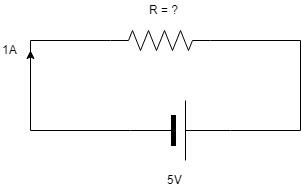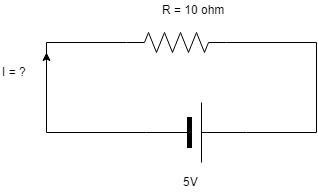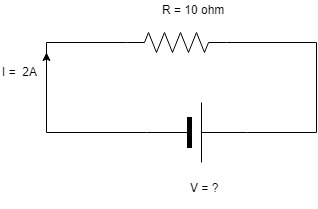# Ohms Law def | Ohms Law with an example problemOhms law def and Ohms Law with an example is shown here.

In other words, it can be called as the voltage is equal to the product of current and Resistance.

If any two parameters are known the other parameter can be calculated by using the following formula.

V = I R

I =V/ R

R = V/ I

where ‘R’ is resistance. The unit of Resistance is Ohm. The reciprocal of resistance is conductance which is called as mho.

‘V’ is the voltage. The unit of Voltage is Volts.

‘I’ is the current. The unit of current is Ampere.

Also, Read: Calculate | Equivalent Resistance

Capacitor classification, application, and drawbacks

The majority of concepts and theorems in electrical and electronics engineering is based on the Ohm’s law.

The following example problem will make you understand Ohms law def better.Here the value of R is unknown. The known values are I and V.

We can find the value of ‘R’ by using the formula R = V/I

R = 5/1

R  = 5 OhmThe Value of I is unknown in the above circuit. The Known values are R and V.

We can find the value of I by using the formula I = V/ R.

I = 5 / 10

I = .5 AThe value of V is unknown here. The known values are I and R.

It can be found by using the formula V = IR.

V = 2 * 10

V = 20v

Also, Read:  KVL and KCL | Kirchoff’s Law

The above ohms law def with an example contains only a single loop (mesh). For the more number of loops and larger circuits, we can’t use this way to find the value of an unknown parameter.  To find the value of unknown parameters in larger circuits we need to use more advanced theorems which we will see in future posts.

To Solve larger circuits we need a scientific calculator to find the solution. Practically, it was much difficult to calculate the value manually. If you don’t have a scientific calculator, try out any mobile apps or any applications which are available online. But I recommend you to use a physical scientific calculator.

Finally, Ohms law def is a basic for all complex problems.

## You may also interested in:

Wind power plant

Thermal Power Plant?

Nuclear Power Plant?

Hydro-electric Power Plant?

Methods of Neutral Grounding | Neutral Earthing